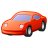# App Development

Discussion board where members can learn more about Qlik Sense App Development and Usage.

Announcements
April 22, 2PM EST: Learn about GeoOperations in Qlik Sense SaaS READ MORE
cancel
Showing results for
Search instead for
Did you mean:Contributor II

## Passing variable - Set Analysis

Hi,

I have created the following variable to be utilized in the set analysis calculation:

vCurrQTR = 2018Q4 (User selected)

Variable Name: vTestQTR

=if(right(vCurrQTR,2) = 'Q4',
chr(39)&left(vCurrQTR,4)&''&'Q1'&chr(39)&chr(44)&
chr(39)&left(vCurrQTR,4)&''&'Q2'&chr(39)&chr(44)&
chr(39)&left(vCurrQTR,4)&''&'Q3'&chr(39)&chr(44)&
chr(39)&left(vCurrQTR,4)&''&'Q4')&chr(39)

If we evaluate the above variable (=vTestQTR) in Text & image object it will yield the following result: '2018Q1','2018Q2','2018Q3','2018Q4'

Currently:
sum({<PERIOD_TYPE = {'Q'},RETURN_TYPE = {'S'},PERIOD = {'2018Q1','2018Q2','2018Q3','2018Q4'}>}Return) gives the correct result.

Now using variable would like to replace the above highlighted condition and get the same result. Unfortunately the variable is not working as expected inside the set analysis expression. Can you help with the correct syntax?
sum({<PERIOD_TYPE = {'Q'},RETURN_TYPE = {'S'},PERIOD = {'variable'}>}Return)

Thanks.

Labels (2)

• ### variable calculation

1 Solution

Accepted SolutionsMVP

Try this

``````if(RETURN_TYPE = 'R', Sum({<PERIOD = {\$(=\$(vTestQTR))}, PERIOD_TYPE = {'Q'}, RETURN_TYPE = {'R'}>} RETURN),
if(RETURN_TYPE = 'S', Sum({<PERIOD = {\$(=\$(vTestQTR))}, PERIOD_TYPE = {'Q'}, RETURN_TYPE = {'S'}>} RETURN),
if(RETURN_TYPE = 'T', Sum({<PERIOD = {\$(=\$(vTestQTR))}, PERIOD_TYPE = {'Q'},RETURN_TYPE = {'T'}>} RETURN)
)))``````
5 RepliesMVP

Try one of these two options

``Sum({<PERIOD_TYPE = {'Q'},RETURN_TYPE = {'S'},PERIOD = {"\$(vTestQTR)"}>} Return)``

Or

``Sum({<PERIOD_TYPE = {'Q'},RETURN_TYPE = {'S'},PERIOD = {"\$(=\$(vTestQTR))"}>} Return)``Contributor II
Author

Thanks for the response. When i tried them its gives me no result.MVP

Would you be able to share a sample where we can see the issue?Contributor II
Author

Sure. The sample file is attached.MVP

Try this

``````if(RETURN_TYPE = 'R', Sum({<PERIOD = {\$(=\$(vTestQTR))}, PERIOD_TYPE = {'Q'}, RETURN_TYPE = {'R'}>} RETURN),
if(RETURN_TYPE = 'S', Sum({<PERIOD = {\$(=\$(vTestQTR))}, PERIOD_TYPE = {'Q'}, RETURN_TYPE = {'S'}>} RETURN),
if(RETURN_TYPE = 'T', Sum({<PERIOD = {\$(=\$(vTestQTR))}, PERIOD_TYPE = {'Q'},RETURN_TYPE = {'T'}>} RETURN)
)))``````